# OzGrid

Excel Charts : Automatically Updating Custom Leader Lines

Category: [Excel]  Demo Available

# Excel Charts: Automatically Updating Custom Leader Lines

Got a Excel Chart question? Use our FREE Excel Help!

###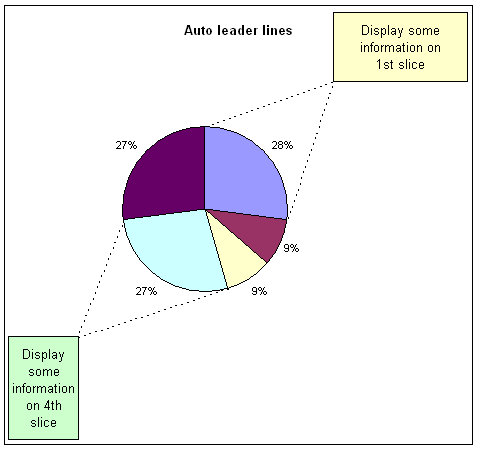This chart has 2 textboxes for displaying information regarding the 1st and 4th slice. The custom leader lines will adjust along with the pie chart data to always connect the textbox corner with the two end points of the pie slice. This effect is created by using a combination chart, pie and xy-scatter, and some math formula.

Below is all the data and formula required to create the chart. The highlighted cells contain the actual charted data.

 A B C D E F 1 Auto leader lines % Angle Radius 2 a 3 0.272727273 98.18182 0.61 3 b 1 0.090909091 32.72727 4 c 1 0.090909091 32.72727 5 d 3 0.272727273 98.18182 6 e 3 0.272727273 98.18182 7 8 9 Slice X Y 10 1 90.00 3.74E-17 0.61 11 0.95 0.95 12 -8.18 0.603791 -0.086812051 13 14 4 -73.64 0.171857 -0.585290714 15 -0.95 -0.95 16 -171.82 -0.60379 -0.086812051

Formulas

 A B C D E F 1 Auto leader lines % Angle Radius 2 a 3 =B2/SUM(\$B\$2:\$B\$6) =360*D2 0.61 3 b 1 =B3/SUM(\$B\$2:\$B\$6) =360*D3 4 c 1 =B4/SUM(\$B\$2:\$B\$6) =360*D4 5 d 3 =B5/SUM(\$B\$2:\$B\$6) =360*D5 6 e 3 =B6/SUM(\$B\$2:\$B\$6) =360*D6 7 8 9 Slice X Y 10 1 90 =COS(RADIANS(B10))*\$F\$2 =SIN(RADIANS(B10))*\$F\$2 11 0.95 0.95 12 =B10-E2 =COS(RADIANS(B12))*\$F\$2 =SIN(RADIANS(B12))*\$F\$2 13 14 4 =90-SUM(E2:E4) =COS(RADIANS(B14))*\$F\$2 =SIN(RADIANS(B14))*\$F\$2 15 -0.95 -0.95 16 =B14-E5 =COS(RADIANS(B16))*\$F\$2 =SIN(RADIANS(B16))*\$F\$2

###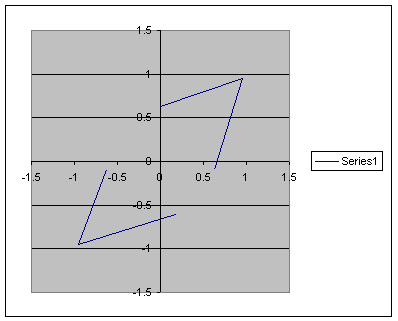Use the source data dialog to add another series to the chart.

###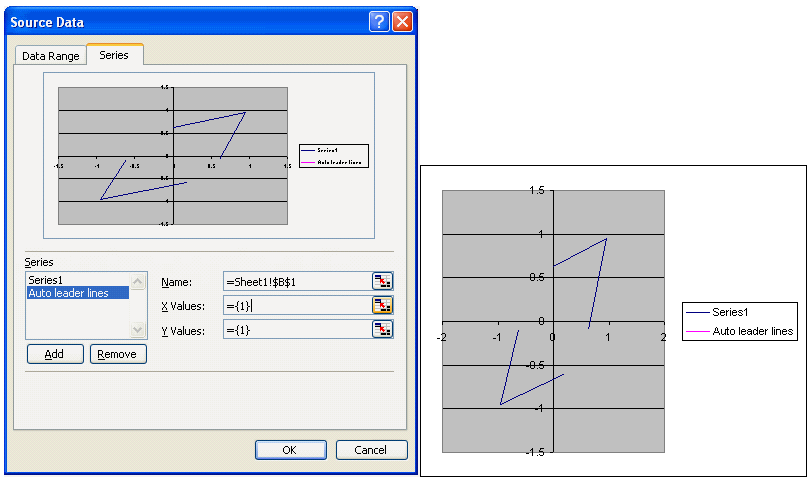Select the 2nd data series and change the chart type to pie.

###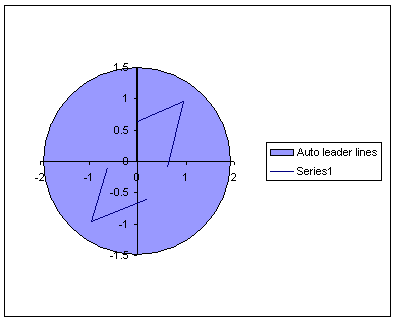Use the source data to specify the location of data, B2:B6, and labels, A2:A6, for the pie chart.

###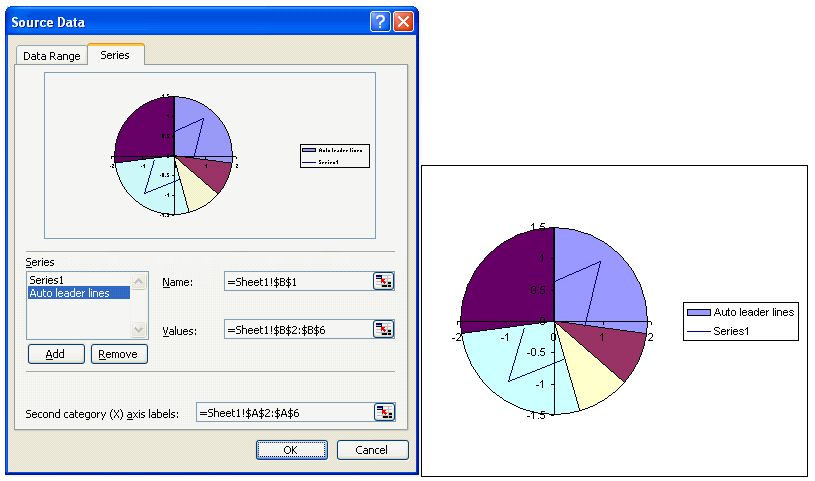Change the axis properties for both x and y axis. Set the minimum and maximum value to -1 and 1 respectively.

###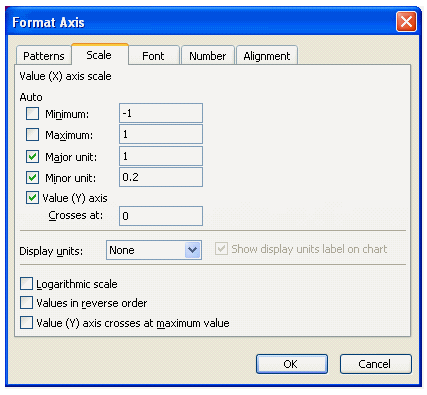The only problem with the chart in its current configuration is that the area available for the leader lines is restricted to the plot area, which is too close to the edge of the pie chart.

###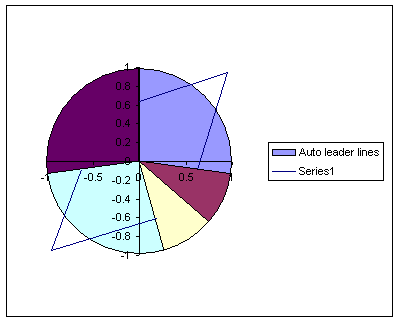To increase this area we need to explode and re assemble the pie chart. Select the pie and drag the slices away from the center. The further away you drag the slices the smaller the pie chart will end up.

###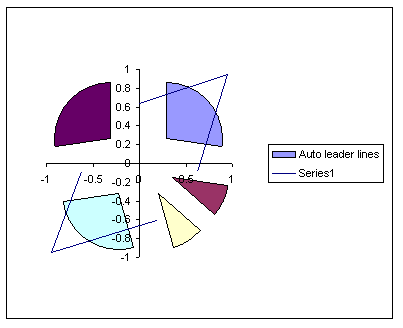After exploding and thereby reducing the size of the pie we need to drag the slice back together. If you do this whilst all the slices are selected then the pie will return to its original size. You have to do each piece individually. So select the pie and then select an individual slice. Drag this back to the center and then repeat for all the other pieces.

###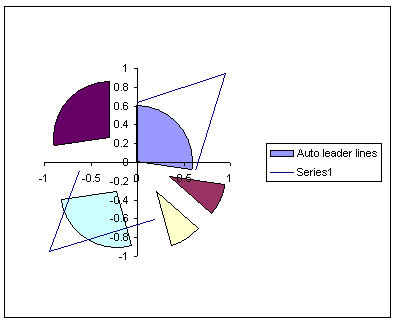You now have a smaller pie chart which will allow more space for the leader lines. The actual radius of the chart can be entered in to the cell F2. The end point of the leader lines, where the 2 lines meet, is controlled by the values used in cells C11:D11 and C15:D15. The position of contact of the leader lines with the pies circumference is calculated via the formulas.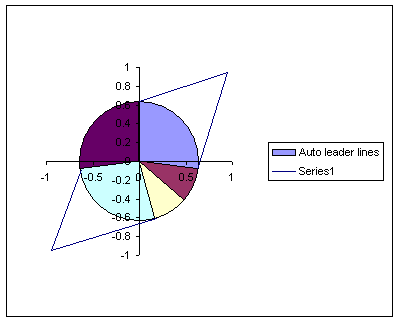Format both axis to remove lines, labels and tickmarks.

###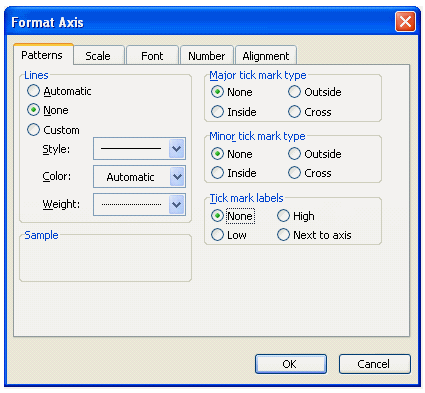You should now have a pie chart with leader lines for slices 1 and 4. This will automatically adjust for any changes in the pie charts data.

###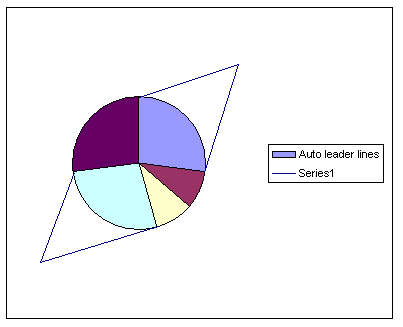Further formatting of the pie, leader lines, legend and chart can be applied. As can the textboxes used to hold any information needed.

Click here to visit our Free 24/7 Excel/VBA Help Forum where there are thousands of posts you can get information from, or you can join the Forum and post your own questions. Escort in Auckland XPAGE

Microsoft ® and Microsoft Excel ® are registered trademarks of Microsoft Corporation. OzGrid is in no way associated with Microsoft.

### Gallery

stars (0 Reviews)